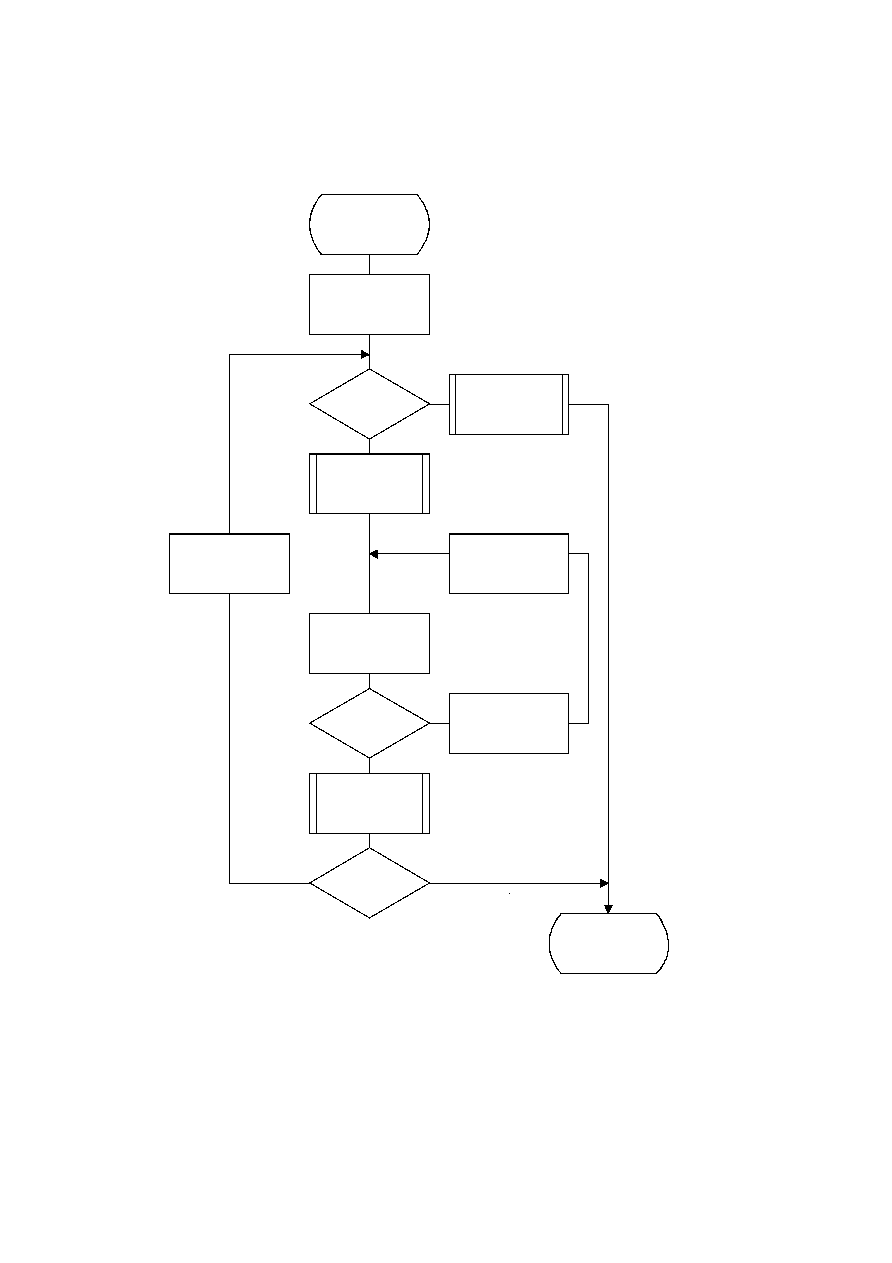ISO/IEC 10918-1 : 1993(E)
There are two significant differences between this sequence and the similar set of operations described in F.1.2 for
Huffman coding. First, the sign is encoded before the magnitude category is identified, and second, the magnitude is
decremented by 1 before the magnitude category is identified.
TISO1370-93/d075
Encode_AC_
Coefficients
K = Kmin
K = EOB
?
Yes
No
Code_1(SE)
Code_0(SE)
K = K + 1
K = K + 1
V = ZZ(K)
V = 0
?
Yes
No
Code_0(S0)
Code_1(S0)
Encode_V(S0)
K = Se
?
Yes
No
Done
Figure F.5 ­ AC coding model for arithmetic coding
Figure F.5 [D75] = 21 cm = 821 %
CCITT Rec. T.81 (1992 E)
95